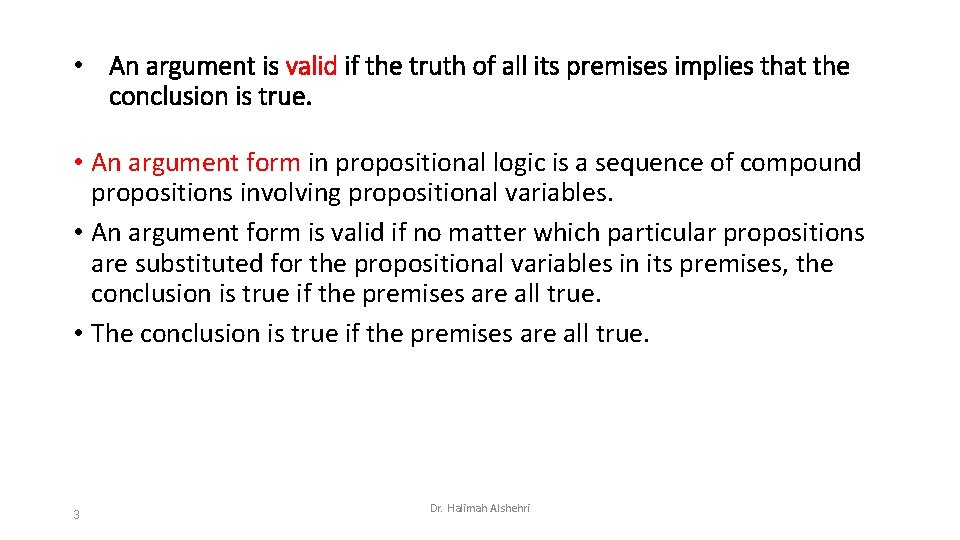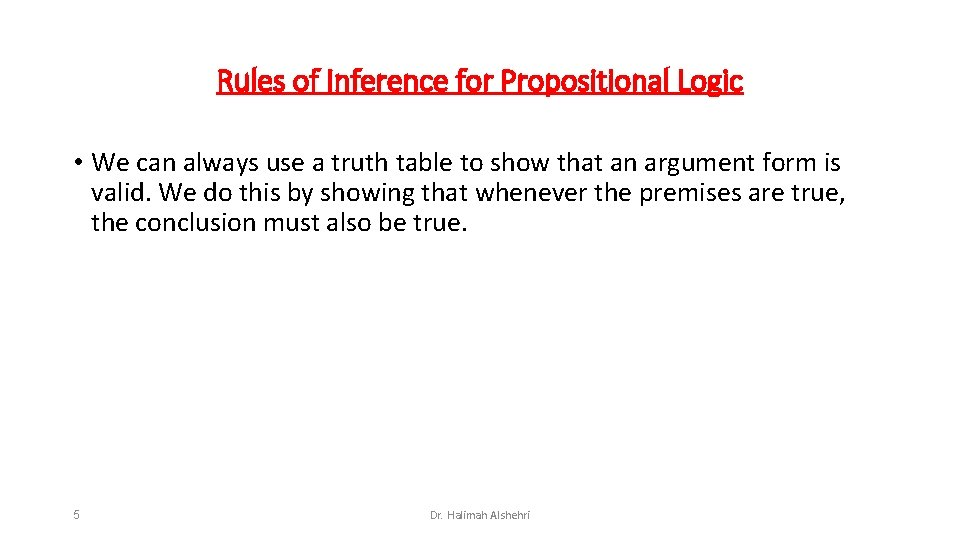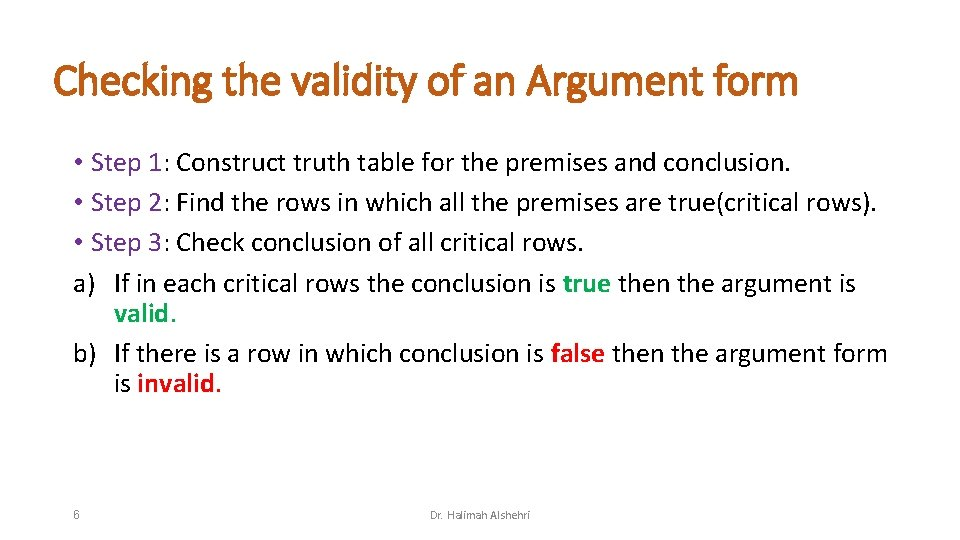# Valid Arguments in Propositional Logic 1 Dr Halimah

• Slides: 9Valid Arguments in Propositional Logic 1 Dr. Halimah AlshehriArgument • An argument in propositional logic is a sequence of propositions. All but the final proposition in the argument are called premises and the final proposition is called the conclusion. Premises ⇒ Conclusion Example: “If you have a current password, then you can log onto the network. ” “You have a current password. ” Therefore, “You can log onto the network. ” 2 Dr. Halimah Alshehri• An argument is valid if the truth of all its premises implies that the conclusion is true. • An argument form in propositional logic is a sequence of compound propositions involving propositional variables. • An argument form is valid if no matter which particular propositions are substituted for the propositional variables in its premises, the conclusion is true if the premises are all true. • The conclusion is true if the premises are all true. 3 Dr. Halimah AlshehriExample: • Either team A or Team B will win the match • Team B lost • Therefore Team A won -----------------------The general form of this argument is: Either P or Q Not P Therefore Q (P ∨ Q) ∧ ┐P→Q , or 4 Dr. Halimah AlshehriRules of Inference for Propositional Logic • We can always use a truth table to show that an argument form is valid. We do this by showing that whenever the premises are true, the conclusion must also be true. 5 Dr. Halimah AlshehriChecking the validity of an Argument form • Step 1: Construct truth table for the premises and conclusion. • Step 2: Find the rows in which all the premises are true(critical rows). • Step 3: Check conclusion of all critical rows. a) If in each critical rows the conclusion is true then the argument is valid. b) If there is a row in which conclusion is false then the argument form is invalid. 6 Dr. Halimah AlshehriExample: Determine whether the following argument is valid or invalid P q ┐q T T F T F F F T T T Solution put all premises true: Informal p→q=T ┐p = T, then p = F Now, put q = T. So, we still have p → q = T, but q = T, Then ┐q = F. The conclusion is False whereas all premises are true, Therefor, the argument is invalid. 7 Dr. Halimah Alshehri FormalExample : Determine whether the following argument is valid or invalid (Hint: use informal method) Solution: put all premises true: (p → q) = T q → (p → r) = T p=T. . . . . Since p = T and (p → q) = T, then q = T Now, since q = T and q → (p → r) = T then Q : = (p → r) = T We have p = T and (p → r) = T then r must be true. Therefore r = T and the argument is valid. 8 Dr. Halimah AlshehriExercise: • 9 Dr. Halimah Alshehri